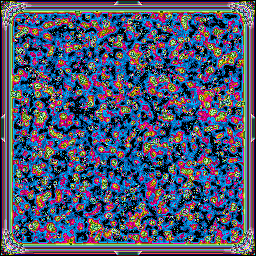• Home
• Files
• If...if...We didn't love freedom enough. And even more, we had no awareness of the real situation.... We purely and simply deserved everything that happened afterward.

# Day 16

Previous: Day 15 | Next: Day 17
###AUTO-ACQUIRED DATA FOLLOWS…
day-16/julia-day16.jl
day-16/julia-day16.jl
```function main(io)
rules, yourticket, tickets = parseinput(io)
silver = partone(rules, tickets)
gold = parttwo(tickets, yourticket, rules)
silver, gold
end

function parseinput(io)
data = read(io, String) |> x->replace(x, "\r"=>"")
fields, yourticket, tickets = split(data, "\n\n")
rules = parserules(fields)
yourticket = parsetickets(yourticket, true)
tickets = parsetickets(tickets, false)
(rules, yourticket, tickets)
end

function torange(x::T) where T <: AbstractString
low, high = split(x, &\#39;-&\#39;)
return parse(Int, low):parse(Int, high)
end

function parserules(data)
rules = Dict()
for line in split(data, "\n"; keepempty=false)
field, ranges = match(r"^(.+): (.+)\$", line).captures
ranges = [torange(rangestr) for rangestr in split(ranges, " or ")]
rules[field] = ranges
end
rules
end

function parsetickets(data, myticket)
split(data, "\n"; keepempty=false)[2:(myticket ? 2 : end)] .|> x -> split(x, &\#39;,&\#39;) .|> x -> parse(Int, x)
end

function partone(rules, tickets)
ranges = [r for r in Iterators.flatten(values(rules))]
\# v = t -> validate(t, ranges)
\# v.(tickets) |> sum
errors = tickets .|> t -> validate(t, ranges)
errors |> sum
end

function validate(ticket, ranges)
for ticketval in ticket
if !any(range->ticketval in range, ranges)
return ticketval
end
end
0
end

function parttwo(tickets, yourticket, rules)
potentialmap = possibilities(append!(yourticket, tickets), rules)
truthmap = mapsieve(potentialmap)
indices = filter(x->startswith(x.first, "departure"), truthmap) |> values |> collect
getindex(yourticket, indices) |> prod
end

function possibilities(alltickets, rules)
ranges = [r for r in Iterators.flatten(values(rules))]

potentialmap = Dict(index => Set(collect(keys(rules))) for index in 1:length(alltickets))
valid\_tickets = filter(t->validate(t,ranges)==0, alltickets)

for ticket in valid\_tickets
for i in eachindex(ticket)
for (key, ranges) in rules
!(ticket[i] in Iterators.flatten(ranges)) && (delete!(potentialmap[i], key))
end
end
end
potentialmap
end

function mapsieve(potentialmap)
solved = Dict()
for (index, locations) in sort(potentialmap, by=x->length(potentialmap[x]))
if length(locations) == 1
known\_loc = pop!(locations)
solved[known\_loc] = index
delete!(potentialmap, index)
for others in values(potentialmap)
delete!(others, known\_loc)
end
end
end
solved
end
```

Tags: